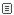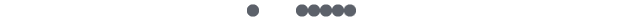Python-集合类型

set 称做由不同的元素组成的集合，集合(set)的成员通常被称做集合元素(set

elements)Python 把这个概念引入到它的集合类型对象里。集合对象是一组无序排列的可哈希的值

(frozenset)则正好相反，即，他们有哈希值，能被用做字典的键或是作为集合中的一个成员。

frozenset():    //创建不可变集合

>>>s=set('cheeseshop')

>>> s

set(['c', 'e', 'h','o', 'p', 's'])

>>>t=frozenset('bookshop')

>>> t

frozenset(['b', 'h','k', 'o', 'p', 's'])

>>> type(s)

<type 'set'>

>>> type(t)

<type'frozenset'>

>>> len(t)

6

>>> 'b' in t

True

>>> 'rsc' in t

False

>>> for i int:

...     print i,

...

b h k o p s

>>> s

set(['c', 'e', 'h','o', 'p', 's'])

>>> s

set(['c', 'e', 'h','o', 'rsc', 'p', 's'])

>>>s.update('pypi')

>>> s

set(['c', 'e', 'i','h', 'o', 'rsc', 'p', 's', 'y'])

>>>s.remove('rsc')

>>> s

set(['c', 'e', 'i','h', 'o', 'p', 's', 'y'])

>>> s

set(['c', 'e', 'i','h', 'o', 'p', 's', 'y'])

>>> del s

>>>s=set('rscpass')

>>> s

set(['a', 'p', 's','r', 'c'])

>>> 'a' in s

True

>>> 'ba' ins

False

>>> t

frozenset(['b', 'h','k', 'o', 'p', 's'])

>>> s

set(['a', 'p', 's','r', 'c'])

>>> s == t

False

>>> s != t

True

“小于符号( <, <= )

>>>set('shop') < set('cheeseshop')

True

>>>set('bookshop') >=set('shop')

True

>>> s

set(['a', 'p', 's','r', 'c'])

>>> t

frozenset(['b', 'h','k', 'o', 'p', 's'])

>>> s | t

set(['a', 'c', 'b','h', 'k', 'o', 'p', 's', 'r'])

intersection()

>>> s

set(['a', 'p', 's','r', 'c'])

>>> t

frozenset(['b', 'h','k', 'o', 'p', 's'])

>>> s &t

set(['p', 's'])

>>> s

set(['a', 'p', 's','r', 'c'])

>>> t

frozenset(['b', 'h','k', 'o', 'p', 's'])

>>> s - t

set(['a', 'c', 'r'])

C,该集合中的元素，只能是属于集合 s 或者集合 t

>>> s

set(['a', 'p', 's','r', 'c'])

>>> t

frozenset(['b', 'h','k', 'o', 'p', 's'])

>>> s ^ t

set(['a', 'c', 'b','h', 'k', 'o', 'r'])

>>> t

frozenset(['b', 'h','k', 'o', 'p', 's'])

>>> s

set(['a', 'p', 's','r', 'c'])

>>> t | s

frozenset(['a', 'c','b', 'h', 'k', 'o', 'p', 's', 'r'])

>>> t &s

frozenset(['p', 's'])

(Union) Update ( |= )

>>> s

set(['a', 'p', 's','r', 'c'])

>>>u=frozenset(s)

>>> u

frozenset(['a', 'p','s', 'r', 'c'])

>>> s|=set('pypi')

>>> s

set(['a', 'c', 'i','p', 's', 'r', 'y'])

>>> s

set(['a', 'p', 's','r', 'c'])

>>> s &= set('shop')

File "<stdin>", line 1

s & = set('shop')

^

SyntaxError: invalidsyntax

>>> s &=set('shop')

>>> s

set(['p', 's'])

len()

>>> len(s)

7

>>> s

set(['a', 'c', 'b','h', 'k', 'o', 'r'])

set() and frozenset()

set() frozenset()工厂函数分别用来生成可变和不可变的集合。

s.issubset(t)  如果st的子集，则返回 True,否则返回 False

s.issuperset(t) 如果ts的超集，则返回 True,否则返回 False

s.union(t)  返回一个新集合，该集合是st的并集

s.intersection(t)  返回一个新集合，该集合是st的交集

s.difference(t) 返回一个新集合，该集合是 s 的成员，但不是 t 的成员

s.symmetric_difference(t)返回一个新集合，该集合是 s t 的成员，但不是st共有的

s.copy() 返回一个新集合，它是集合 s 的浅复制

s.update(t)  t 中的元素修改 s, 即，s 现在包含st的成员

s.intersection_update(t)  s 中的成员是共同属于st的元素。

s.difference_update(t)  s 中的成员是属于 s 但不包含在 t 中的元素

s.symmetric_difference_update(t)s 中的成员更新为那些包含在st中，但不 s

t 共有的元素

s.remove(obj)  从集合 s 中删除对象 obj；如果 obj 不是集合 s 中的元素(obj not

in s)，将引发 KeyError 错误

s.discard(obj)  如果 obj 是集合 s 中的元素，从集合 s 中删除对象 obj

s.pop()   删除集合 s 中的任意一个对象，并返回它

s.clear()  删除集合 s 中的所有元素

7.6 集合类型操作符、函数和方法

len(s)  集合基数: 集合 s 中元素的个数

set([obj])  可变集合工厂函数; obj 必须是支持迭代的，由 obj

frozenset([obj])  不可变集合工厂函数; 执行方式和 set()方法相同，

obj in s 成员测试：obj s 中的一个元素吗?

obj not in s  非成员测试：obj 不是 s 中的一个元素吗?

s == t  等价测试: 测试st是否具有相同的元素?

s != t  不等价测试: ==相反

s < t  (严格意义上)子集测试; s != t 而且 s

s.issubset(t) s <=t  子集测试(允许不严格意义上的子集): s 中所有的元素

s > t  (严格意义上)超集测试: s != t 而且 t 中所有的元素

s.issuperset(t) s>= t  超集测试(允许不严格意义上的超集): t 中所有的元素

s.union(t)  s | t 合并操作：s t 中的元素

s.intersec- tion(t) s& t 交集操作：s t 中的元素

s.difference(t) s -t  差分操作: s 中的元素，而不是 t 中的元素

s.symmetric_difference(t)s^ t  对称差分操作：s t 中的元素，但不是st共有

s.copy() 复制操作:返回 s (浅复制)副本

s.update(t)  s |= t (Union) 修改操作: t 中的成员添加 s

s.intersection_update(t)s &= t  <span style="font-fa评论发表评论